# Verilog 学习笔记（7）——应用：线性进位选择加法器Contents

## 应用：线性进位选择加法器

### 基本原理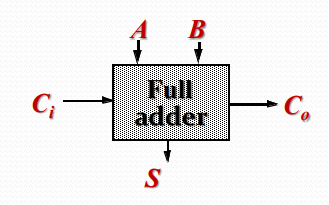$A,B$ 为两个输入，$C_i$ 为上一级进位输入，$S$ 为该位和，$Co$ 为进位输出。

$$S=A\oplus B\oplus C\\ C_o=AB+BC_i+AC_i$$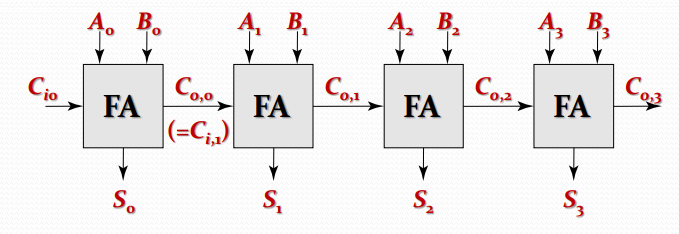$$t_{adder}=(N-1)t_{carry}+t_{sum}$$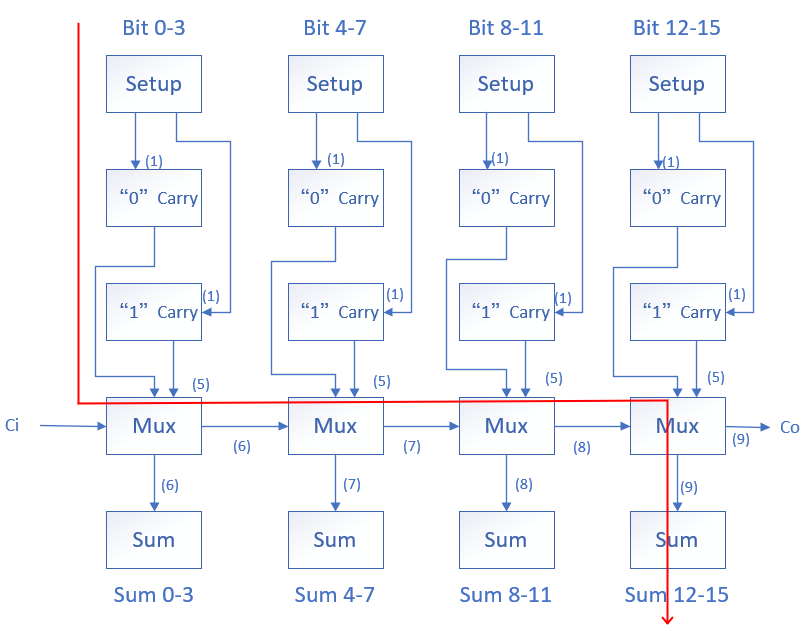$$t_{adder}=t_{setup}+M\cdot t_{carry}+N/M \cdot t_{mux}+t_{sum}$$

### Verilog 部分

#### 进位输入为 0 的 4 位全加器

module adder4_c0(
input [3:0] a,
input [3:0] b,
output [4:0] sum
);

assign sum=a+b;

endmodule


#### 进位输入为 1 的 4 位全加器

module adder4_c1(
input [3:0] a,
input [3:0] b,
output [4:0] sum
);

assign sum=a+b+1;

endmodule


#### 单级 4 位进位选择加法器

module adder4(
input [3:0] a,
input [3:0] b,
input cin,
output [3:0] sum,
output cout
);

wire [4:0] sum0,sum1;

.a(a),
.b(b),
.sum(sum0)
);

.a(a),
.b(b),
.sum(sum1)
);

assign {cout,sum}=cin?sum1:sum0;

endmodule


#### 16 位线性进位选择加法器 (top_module)

module adder16(
input [15:0] a,
input [15:0] b,
input cin,
output [15:0] sum,
output cout
);

wire [2:0] cp;

.a(a[3:0]),
.b(b[3:0]),
.cin(cin),
.sum(sum[3:0]),
.cout(cp)
);

.a(a[7:4]),
.b(b[7:4]),
.cin(cp),
.sum(sum[7:4]),
.cout(cp)
);

.a(a[11:8]),
.b(b[11:8]),
.cin(cp),
.sum(sum[11:8]),
.cout(cp)
);

.a(a[15:12]),
.b(b[15:12]),
.cin(cp),
.sum(sum[15:12]),
.cout(cout)
);

endmodule


#### testbench

AB进位
000000000000
eeef00010001
ddfd00100010
ddfe00020011
cdcc03000100
cdcb03050101
cdfd02100110
cdfe02020111
a00061111000
aeef60011001
9dfd70101010
8dfe80021011
8dcc73001100
8dcc73041101
8dfd72101110
8dfe72021111

(16 进制表示)

A,B 结构上对称，故不再对换进行测试

timescale 1ns/1ps

reg  [15:0]  A;
reg  [15:0]  B;
wire [16:0]  sum;

initial begin
A = 0;
B = 0;
#10;
A = 16'heeef;
B = 16'h0001;
#10;
A = 16'hddfd;
B = 16'h0010;
#10;
A = 16'hddfe;
B = 16'h0002;
#10;
A = 16'hcdcc;
B = 16'h0300;
#10;
A = 16'hcdcb;
B = 16'h0305;
#10
A = 16'hcdfd;
B = 16'h0210;
#10
A = 16'hcdfe;
B = 16'h0202;
#10
A = 16'ha000;
B = 16'h6111;
#10
A = 16'haeef;
B = 16'h6001;
#10
A = 16'h9dfd;
B = 16'h7010;
#10
A = 16'h8dfe;
B = 16'h8002;
#10
A = 16'h8dcc;
B = 16'h7300;
#10
A = 16'h8dcc;
B = 16'h7304;
#10
A = 16'h8dfd;
B = 16'h7210;
#10
A = 16'h8dfe;
B = 16'h7202;
end

.a(A),
.b(B),
.cin(1'b0),
.sum(sum[15:0]),
.cout(sum)
);

endmodule
`

#### 测试波形结果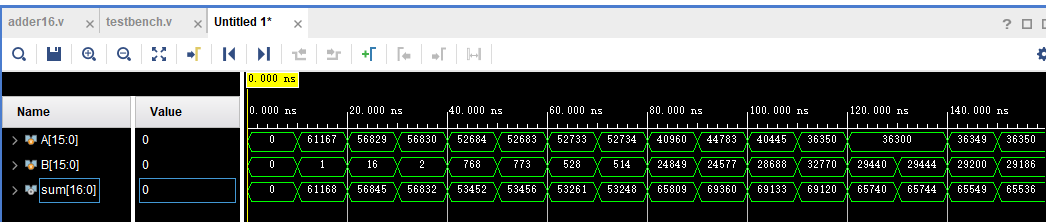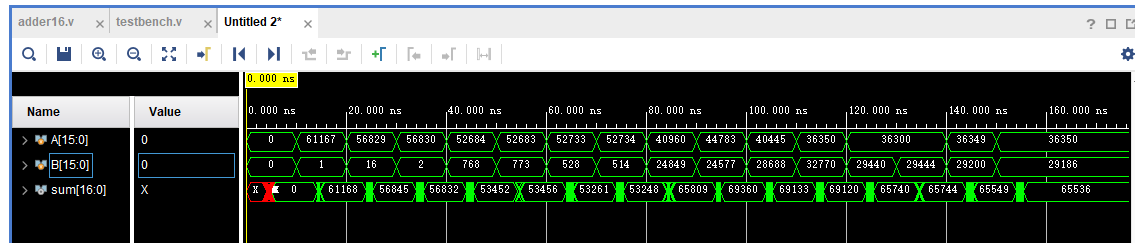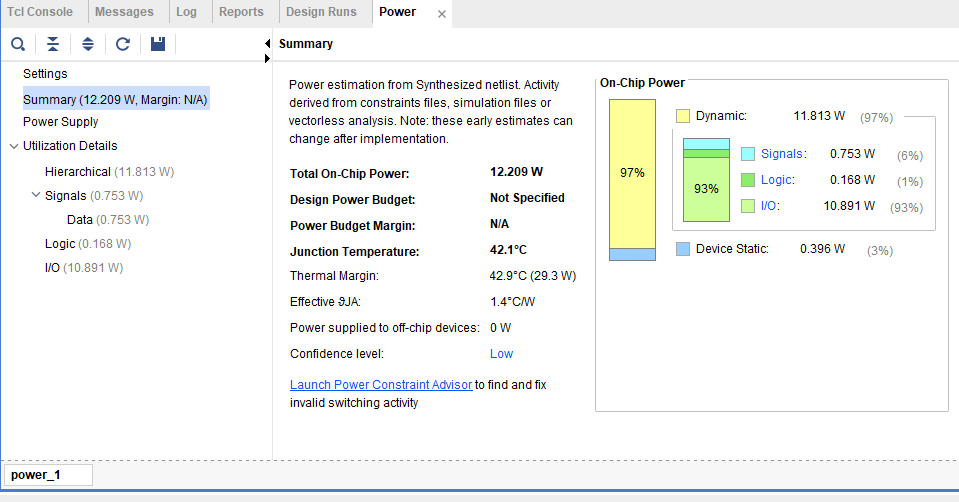1.DylanOuO说道：